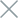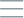• Home
• Tags
• Series
•# Common Functions in PHP - Part 2

### isset() function

Checks if a variable is set and has a value.

``````\$name = "John";
if (isset(\$name)) {
echo "Variable 'name' is set.";
} else {
echo "Variable 'name' is not set.";
}
``````

### empty() function

Checks if a variable is empty or does not exist.

``````\$email = "";
if (empty(\$email)) {
echo "Email is not provided.";
} else {
echo "Email is provided.";
}
``````

### exit() or die() function

Stops the execution of the program and displays a message if needed.

``````\$age = 15;
if (\$age < 18) {
echo "You are not old enough to access.";
exit();
}
echo "Welcome to the website.";
``````

### continue control structure

Skips the current iteration of a loop and moves to the next iteration.

``````for (\$i = 1; \$i <= 10; \$i++) {
if (\$i == 5) {
continue;
}
echo \$i . " ";
}
// Output: 1 2 3 4 6 7 8 9 10
``````

### break control structure

Terminates a loop or the current execution.

``````\$num = 1;
while (true) {
echo \$num . " ";
if (\$num == 5) {
break;
}
\$num++;
}
// Output: 1 2 3 4 5
``````

### var_dump() function

Function is used to display detailed information about a variable or value. It allows you to see the data type, value, and size of the variable.

``````\$number = 10;
\$string = "Hello";
\$array = [1, 2, 3];

var_dump(\$number); // int(10)
var_dump(\$string); // string(5) "Hello"
var_dump(\$array); // array(3) { => int(1) => int(2) => int(3) }
``````

### print() function

Function is used to display a value on the screen. It is similar to `echo`, but it returns a value of `1` if successful.

``````\$name = "John";

print "Hello, " . \$name; // Hello, John
``````

### print_r() function

Function is used to display information about a variable or array in a readable format. It is useful when you want to see the structure and values of an array.

``````\$array = [1, 2, 3];

print_r(\$array);
/* Output:
Array
(
 => 1
 => 2
 => 3
)
*/
``````

Lưu ý: The `var_dump`, `print` and `print_r` functions are often used for debugging purposes, as they do not return a value and only display information on the screen.